# PHY 2049 Physics II Coulombs law n Two

• Slides: 17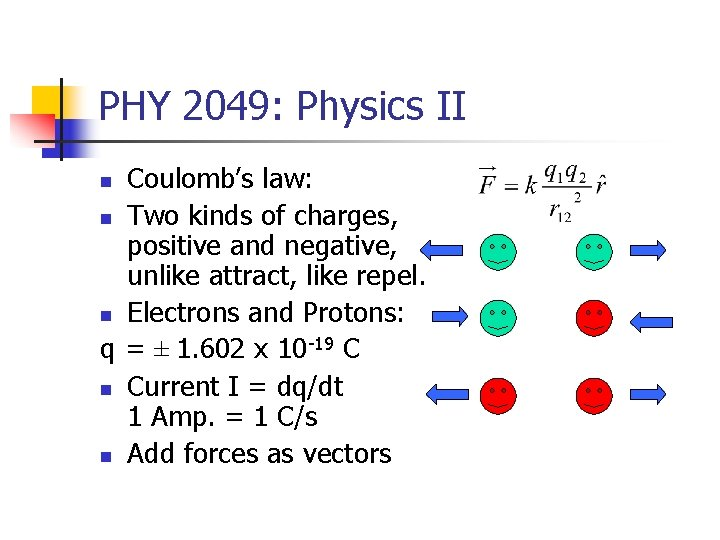PHY 2049: Physics II Coulomb’s law: n Two kinds of charges, positive and negative, unlike attract, like repel. n Electrons and Protons: q = ± 1. 602 x 10 -19 C n Current I = dq/dt 1 Amp. = 1 C/s n Add forces as vectors n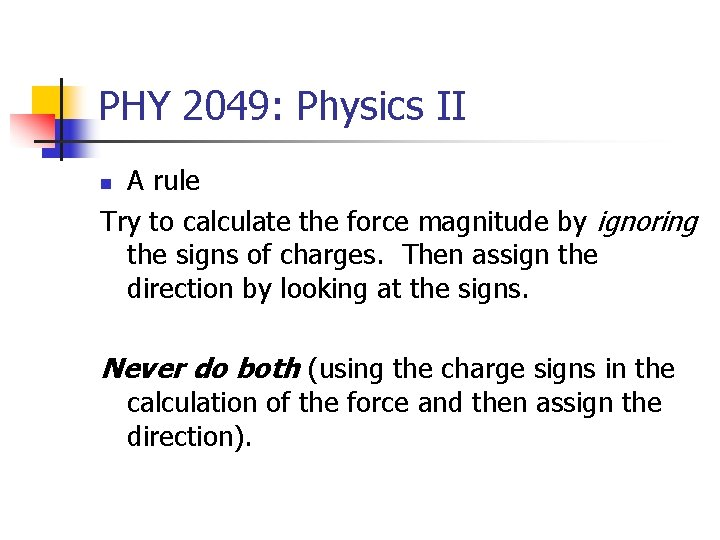PHY 2049: Physics II A rule Try to calculate the force magnitude by ignoring the signs of charges. Then assign the direction by looking at the signs. n Never do both (using the charge signs in the calculation of the force and then assign the direction).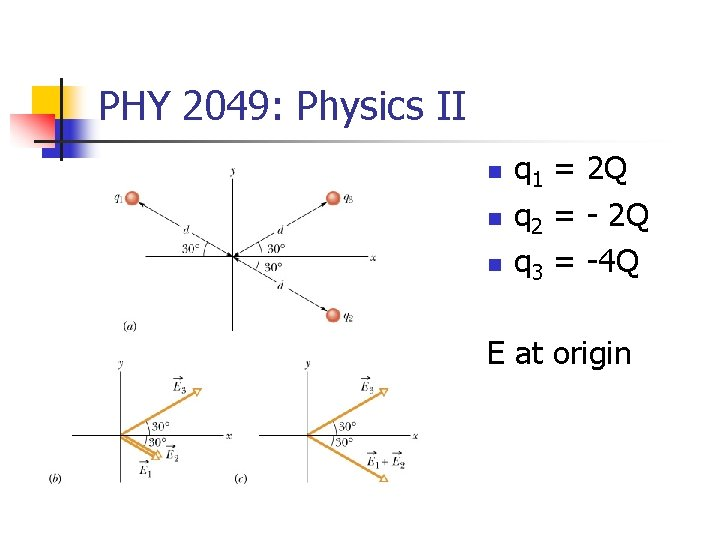PHY 2049: Physics II n n n q 1 = 2 Q q 2 = - 2 Q q 3 = -4 Q E at origin[-0. 344 N, -0. 218 N], 212. 38º Phy 2049: Physics II trial run In Fig. at right, the particles have charges q 1 = -q 2 = 300 n. C and q 3 = -q 4 = 200 n. C, and distance a = 4. 0 cm. n What is the direction of the force on particle 4? A. B. C. D.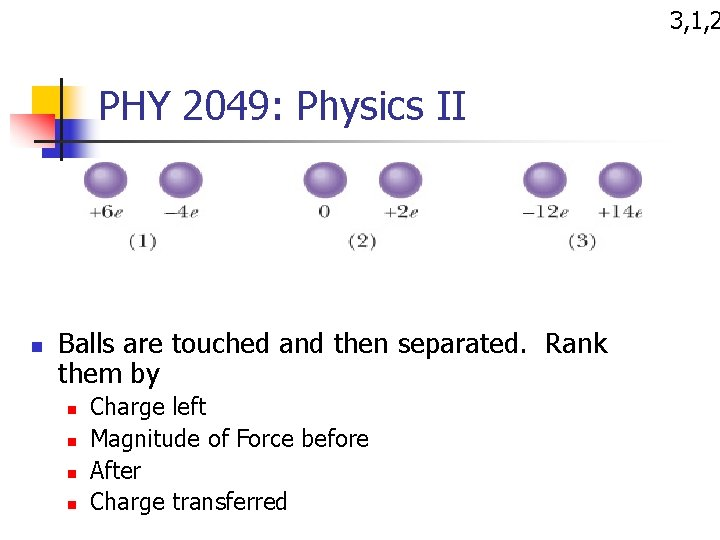3, 1, 2 PHY 2049: Physics II n Balls are touched and then separated. Rank them by n n Charge left Magnitude of Force before After Charge transferredTALP: Take a look Problem PHY 2049: Physics II n n d Force on the center charge = 6 kq 2/d 2 Direction: -x, 180º,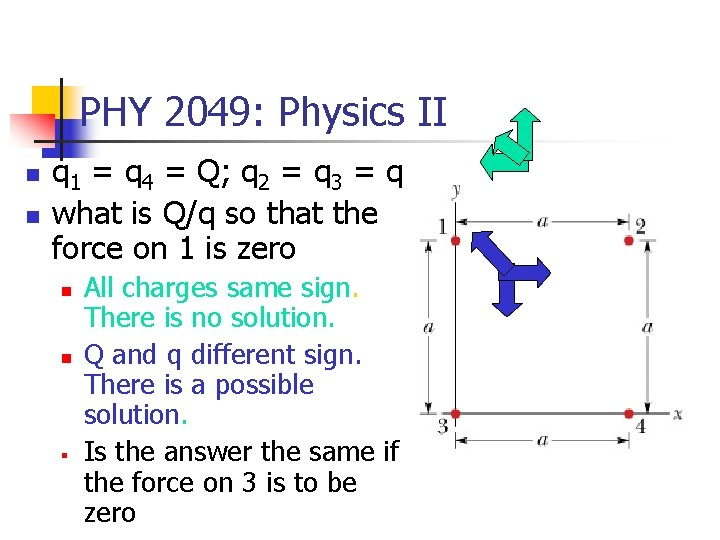PHY 2049: Physics II n n q 1 = q 4 = Q; q 2 = q 3 = q what is Q/q so that the force on 1 is zero n n § All charges same sign. There is no solution. Q and q different sign. There is a possible solution. Is the answer the same if the force on 3 is to be zeroPHY 2049: Physics II n n What is the force on the central chlorine ion? Suppose that we put in an electron that sits on a cesium site and neutralizes it, which way does the Chlorine move?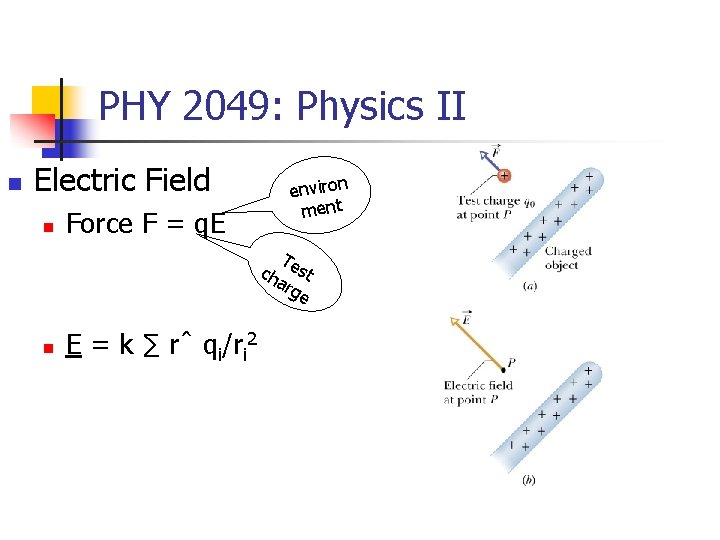PHY 2049: Physics II n Electric Field n Force F = q. E e n vi r o n m en t T c h est a rg e n E = k ∑ rˆ qi/ri 2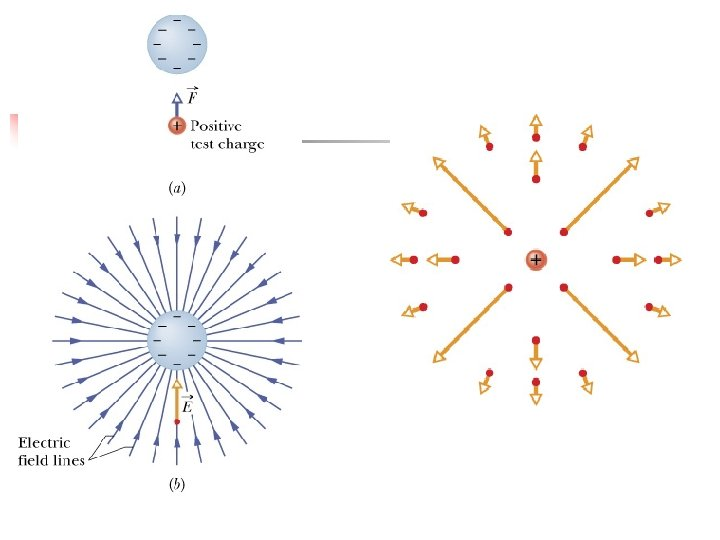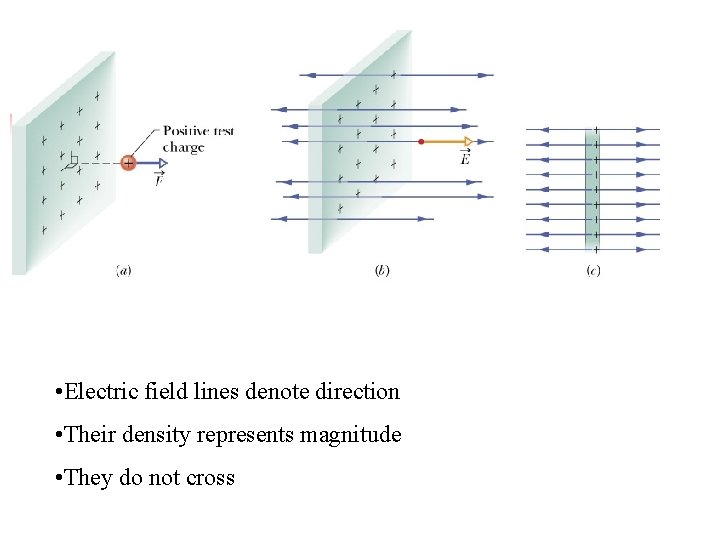• Electric field lines denote direction • Their density represents magnitude • They do not cross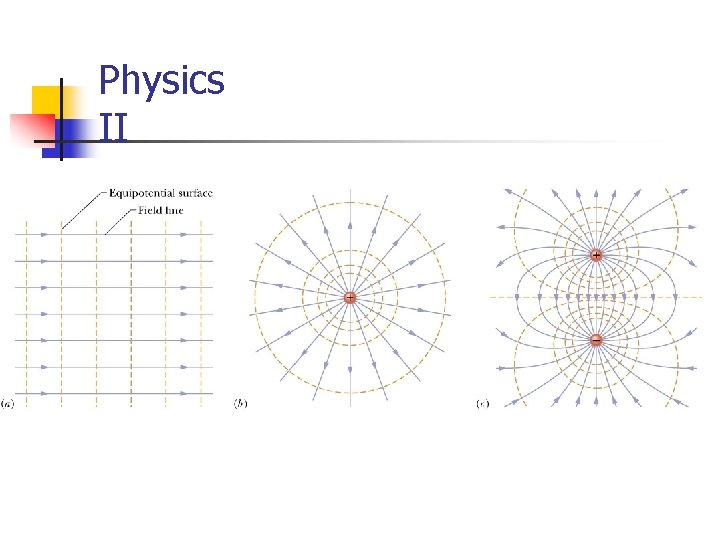Physics II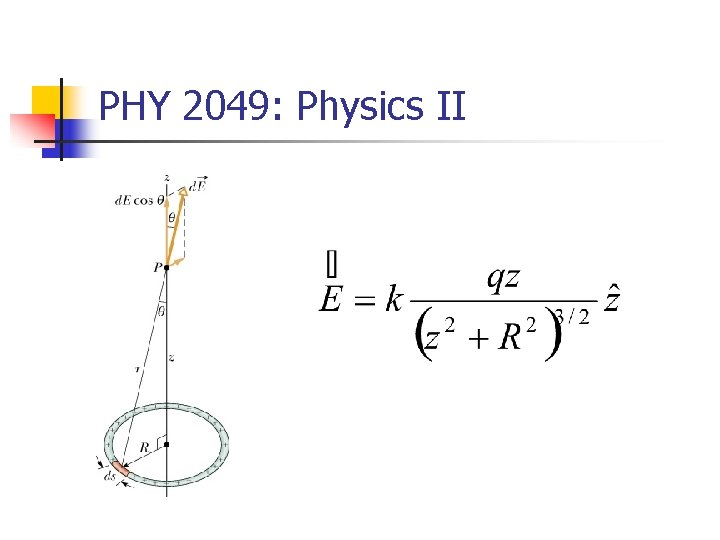PHY 2049: Physics IIPHY 2049: Physics II Induction and charge transfer on contact Forces summed as vectors Electric fieldsPHY 2049: Physics IIPhysics II • Potentials add like numbers